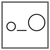Fuddlepixel coz tech is fun();

# Interesting Stuff using Python Part 1

Let us discuss some cool tips and tricks that can be done using Python.

By Khushwant Soni
30 January 2018 · 4 mins read
# `python`In this article, we’re going to do some interesting and fun stuff using python and its built-in Functions that you probably didn’t know about.

• I will be giving all the examples in python v3.6.4
• I am assuming you’re familiar with the basics of python (at least syntax).
• I recommend you to actually code the stuff below on your machines for a better understanding.
• Consider these as tips or tricks to code more easily and conveniently.

## Building a Calculator in Python

Things You Already Need To Know:

• Taking inputs from user
• Working of while loop
• Conditional Statements (If-else)

In this program, we will be building a powerful calculator in which you can give input with any number of operations in the form of any mathematical expression. And we will be also performing calculations on the answers of every step as we do in a real calculator.

You should know about the precedence order of the operations to solve complex expressions, If you don’t know, please refer to the table below (priority From high to low).

Operation Symbol
Parentheses ()
Exponentiation **
Multiplication *
Division /
Subtraction -

Now let’s come to the practical part. Python has a built-in function which you may haven’t used yet, eval(). Eval function takes a string of mathematical expression as input and generates output. Make sure to pass a valid mathematical expression which you want to solve.

You can even pass a string with 500-1000 operands.

Here’s the code of calculator below:

``````print("Enter any expression you want to solve.")
total =''
while True:
ex=input(total)
total = str(eval(total+ex))
choice = input('Do You wish to continue calculating? (Y/N) ')
if (choice == 'n') or (choice == 'N'):
break
else:
continue
``````

Output:

``````Enter any expression you want to solve.
3+6-225**3*5-23//6
Do You wish to continue calculating? (Y/N) Y
-56953119+89**5+7*2
Do You wish to continue calculating? (Y/N) Y
5527106344-7**12-8*9
Do You wish to continue calculating? (Y/N) Y
-8314180929+6**17+9-4//2
Do You wish to continue calculating? (Y/N) N
``````

## Membership Operator ‘in’

Things You Already Need To Know:

• Simply printing statements
• Making data structures like string, list, set, tuple & dictionary.

Most of the beginners use this membership operator (in) only in for loops, But we can also use it directly on data structures like string, list, set, tuple & dictionary. Just write the element and use ‘in’ along with the data structure you want to check into. It will give output in boolean form, Either True or False.

Example 1:

``````mystring='principle'
print('p' in mystring)
``````

Output:

``````True
``````

Example 2:

``````mylist=['p' , 'i' , 'n' , 'e' , 'a' , 'p' , 'p' , 'l' , 'e']
print('i' in mylist)
``````

Output:

``````True
``````

Example 3:

``````myset={ 'p' , 'y' , 't' , 'h' , 'o' , 'n' }
print('k' in myset)
``````

Output:

``````False
``````

Example 4:

``````mytuple=('p','i','n','e','a','p','p','l','e')
print('l' in mytuple)
``````

Output:

``````True
``````

Example 5:

``````mydict={'d':3, 'i':2 , 'n':4, 'e':6, 'a':1, 'p':5, 'l':8}
print('b' in mydict)
``````

Output:

``````False
``````

## Checking Inequalities

Things You Already Need To Know:

• Simply printing statements
• Variables
• Comparison Operators
• Arithmetic Operators

You can check for any inequality in python quite easily. Just write the inequality you want to check and print it. This thing is much useful for large calculations and exponents. The output will be in boolean form, either True or False
Below are the examples containing some of the cases of inequalities.

Example 1:
Consider a very basic inequality with 2 operands

``````var=3
print(1<var)
``````

Output:

``````True
``````

Example 2:
Now we’ll be having 3 operands

``````var=97
print(17<=var<=20)
``````

Output:

``````False
``````

Example 3:
Now it’s time for some exponents.

``````var=113
print(2**4<var<=8**9)
``````

Output:

``````True
``````

Example 4:
Now let’s make a complex inequality

``````var1=87
var2=8**2
var3=15387**5
var4=52*6
print(var3>=var4>=var1>=var2)
``````

Output:

``````True
``````

## Interactive ‘_’ Operator

Things You Already Need To Know:

• Simply printing statements
• working on interactive command line mode

Many of you may haven’t heard of this before. We can check the value of last command executed in interactive command line mode using underscore(’ _ ‘) operator.

Example:

``````>>>"hello"
hello
>>>_
hello
``````

This is only used in the interactive command line of python.

## Printing all the List elements along with their indexes

Things You Already Need To Know:

• Simply printing statements
• For Loop
• Creating a List

At some point of time, you may need to know all the elements present in a list along with their indexes. This code below will get your work done.

``````a=["Apple", "Banana", "Pineapple", "Mango", "Watermelon"]
for i in range(len(a)):
print(a[i],":",i)
``````

Output:

``````Apple : 0
Banana : 1
Pineapple : 2
Mango : 3
Watermelon : 4
``````

##### Update me weekly ✉

Subscribe below and we’ll send you a weekly email summary of all new Code tutorials. Never miss out on learning about the next big thing.

#### Khushwant SoniHe eats and drinks python. Dedicated more than 3 years to study python, Khushwant knows what this language is supposed to do.

Meet me!

#### RecentTop